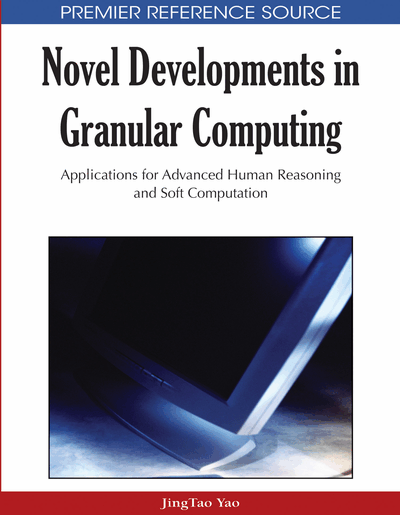# Semantic Analysis of Rough Logic

Qing Liu
DOI: 10.4018/978-1-60566-324-1.ch011
OnDemand:
(Individual Chapters)
Available
\$37.50
No Current Special Offers

## Abstract

In this Chapter, we analyse the semantics of the rough logic. Related operations and related properties of semantics based on the rough logic are also discussed. Related reasoning of the semantis are also studied. Significant of studying semantics of the rough logic will hopefully offer a new idea for the applications to classical logic and other nonstandard logic, also hopefully offer a new theory and methodology for problem resolving in artificial intelligence.
Chapter Preview
Top

## 1. Introduction

Senmantic analysis of logic is a means of studying logic. Kripks tried to study modal logic through Semantic Analysis of Modal Logic, Luis try also to define semantics of Modal Logic as a subset of state space, to study the resolution of Modal Logic by semantics of modal logic. Zadeh tried to change his research from Fuzzy Logic to its semantic, he defined the meaning of fuzzy propositional logical formula as a set of elements of satisfying this fuzzy propositional logical formula in 1979. In this Chapter, we analyse related properties and approximate reasoning of the rough logical semantics. Pawlak proposed Rough Sets based on the semantics of indiscernibility relation, to define the upper and the lower approximations of a undefinable set, so Pawlak’s rough set approach solved the problem of computing elements on boundary by Frege in 1914. From the view of problem solving in artificial intelligence, Hobbs tried to define indiscernibility relation based on the semantics of predicates in logical formulae, he offer a new theoretical tool and methodology for problem solving in artificial intelligence.

In the Chapter, rough logic is described in Section 2. Truth values of the rough logical formulae are defined in Section 3. Sematic model of the rough logic is described in Section 4. Satisfiability of meaning based on rough logical formulae is also described in Section 5. The Related operations of semantics based the rough logic are presented in Section 6. Related properties of semantics based on the rough logic are given in Section 7. The normal forms of meaning of rough logical formulae are discussed in Section 8. Reasoning based on semantics of rough logical formula is discussed in Section 9. Applications of the semantics based on rough logic are presented in Section 10. Final Section is the perspective of studying the semantics of rough logic.

## Complete Chapter List

Search this Book:
Reset